# Mixed Effects Models - Random Intercepts

Jul. 2, 2021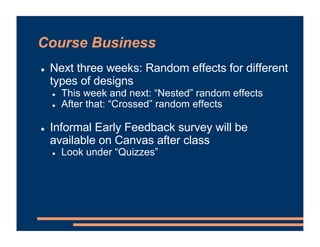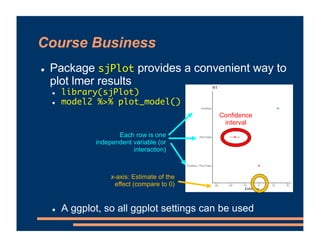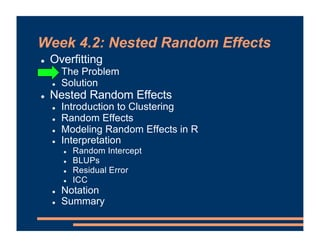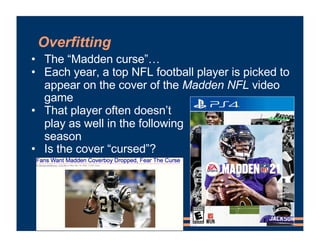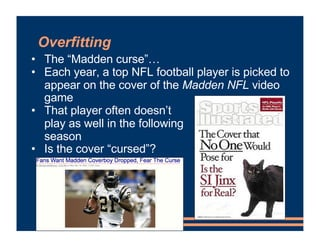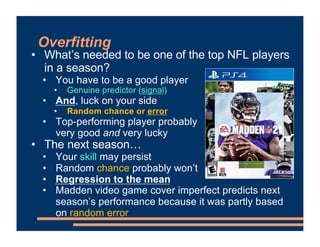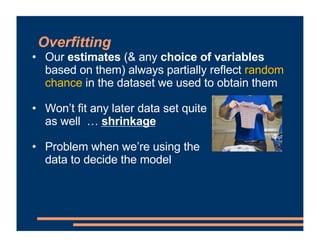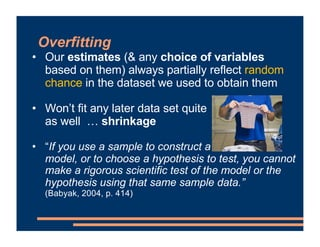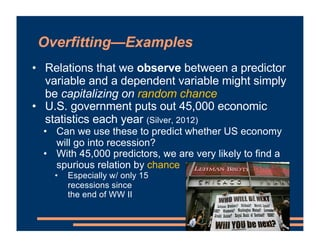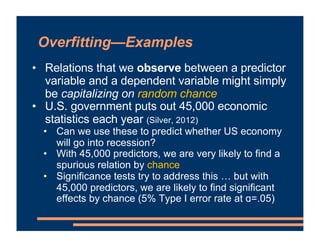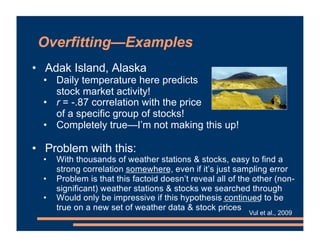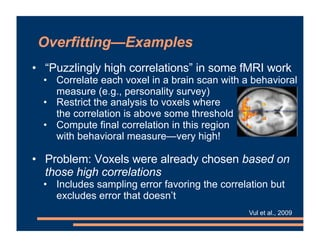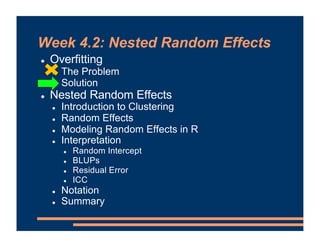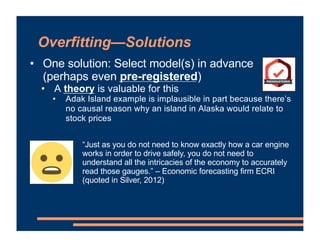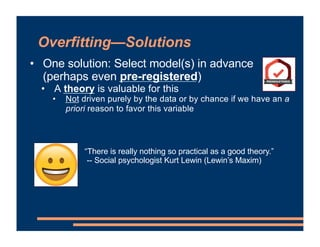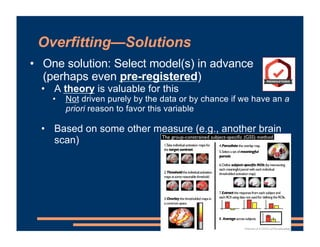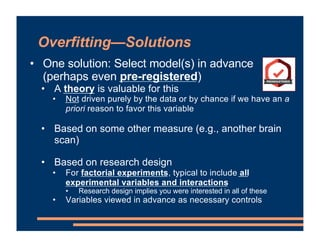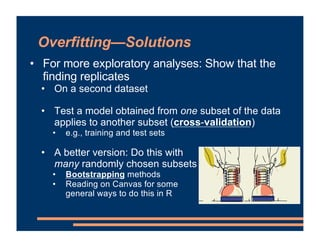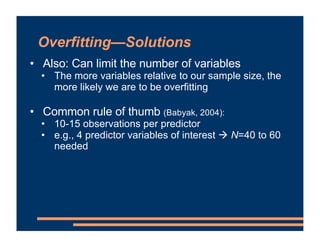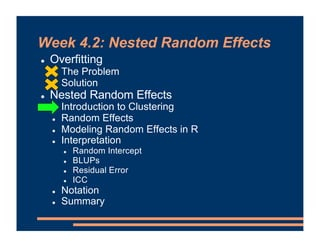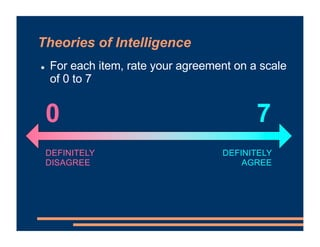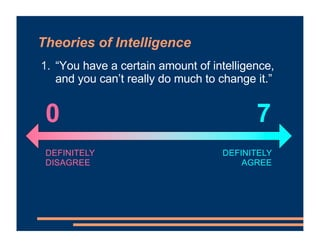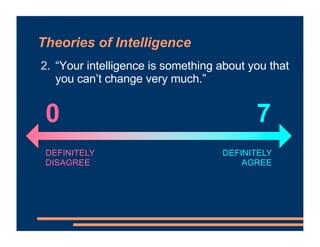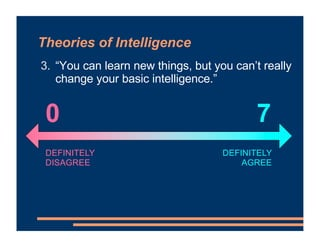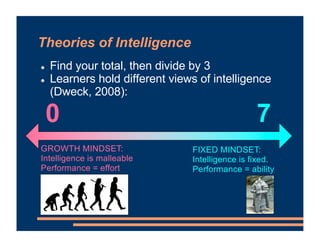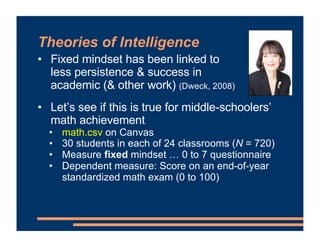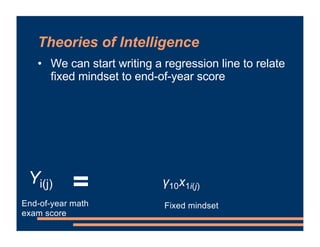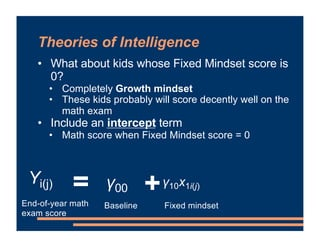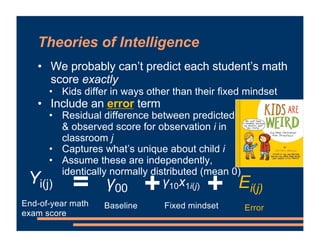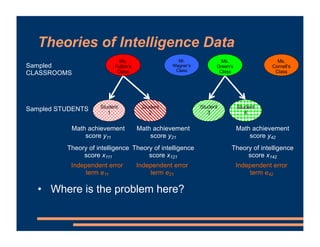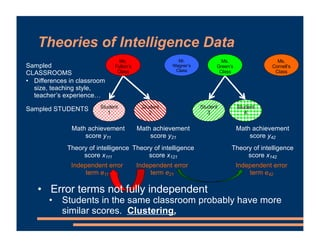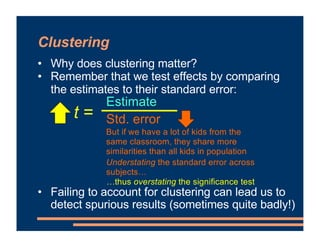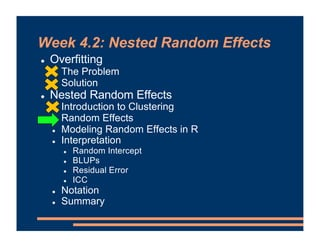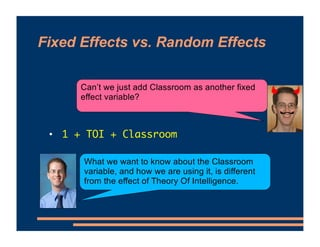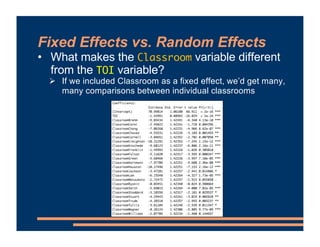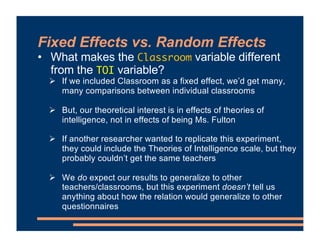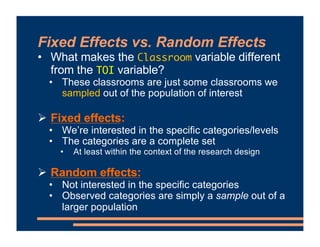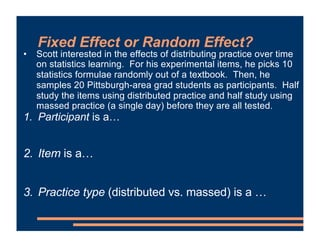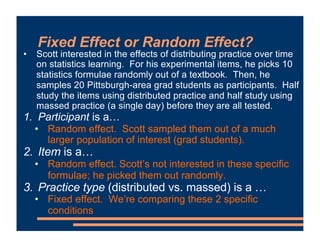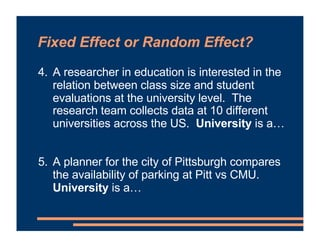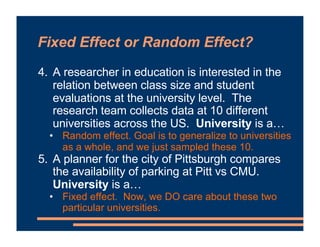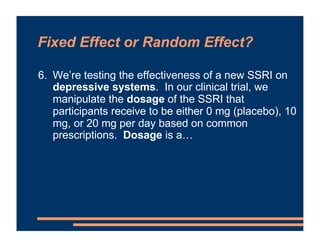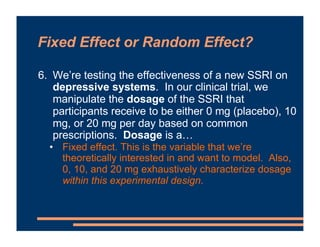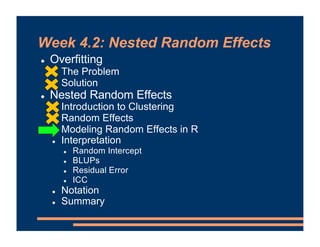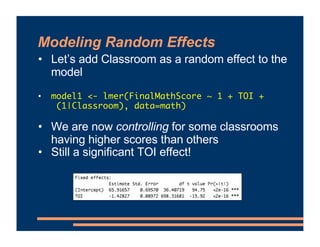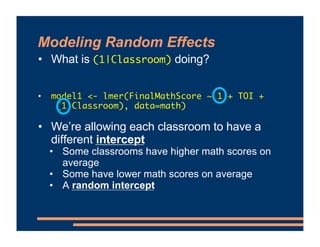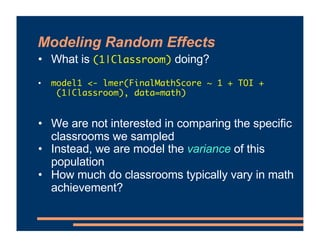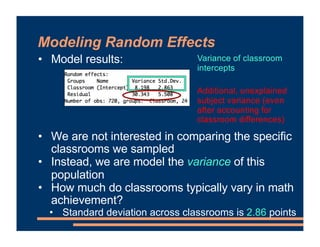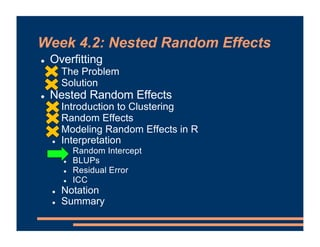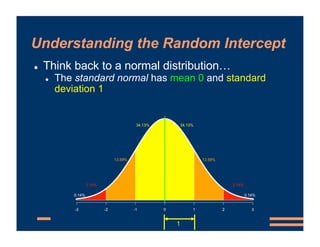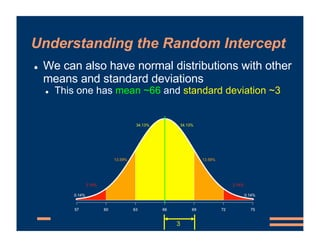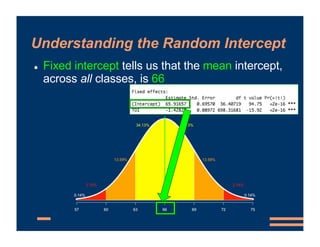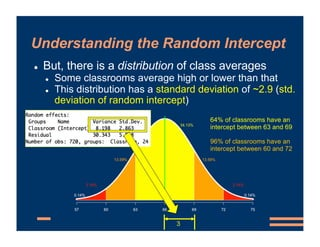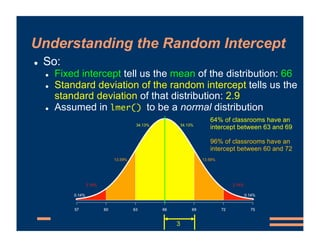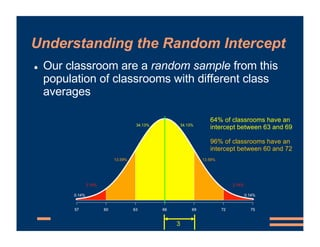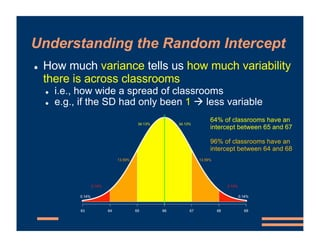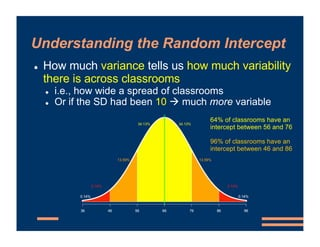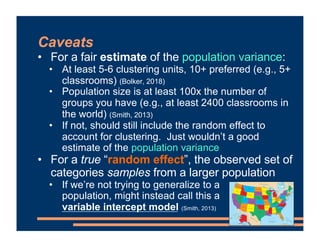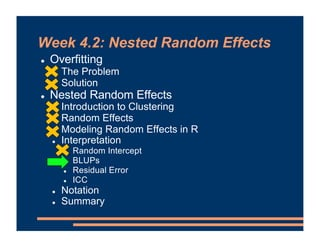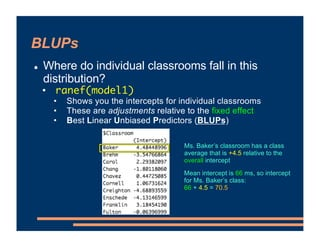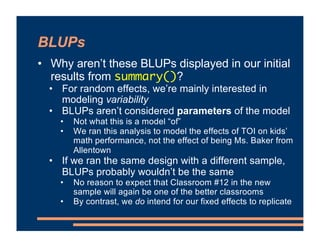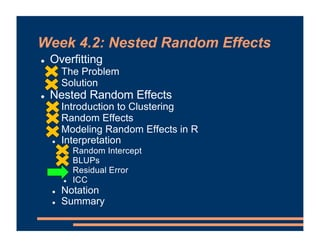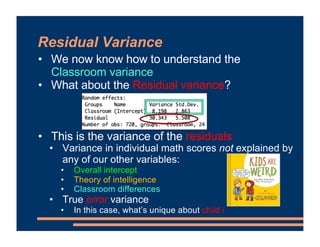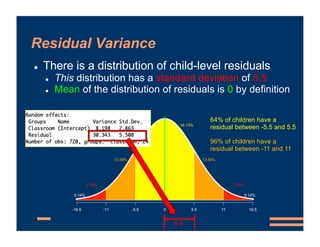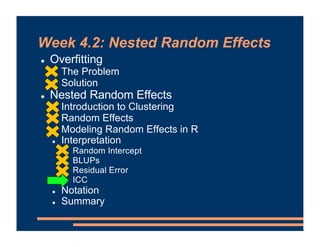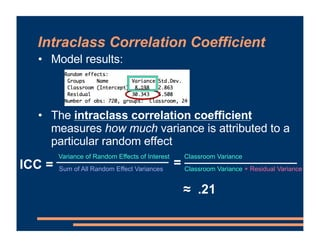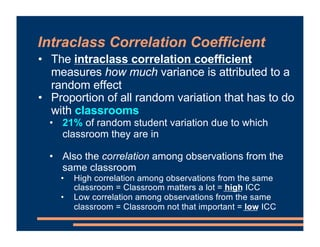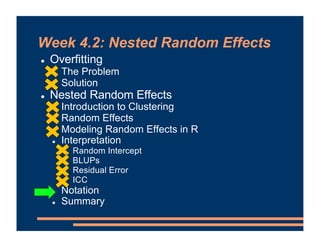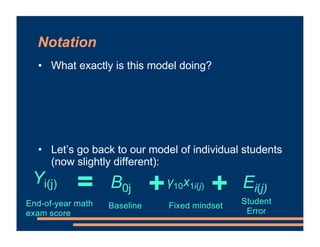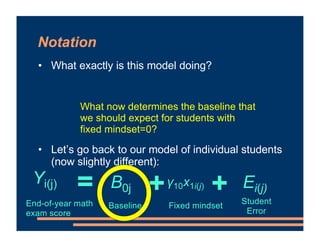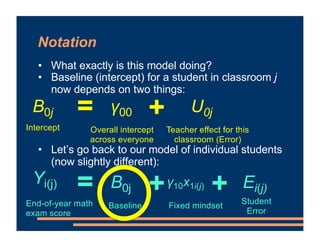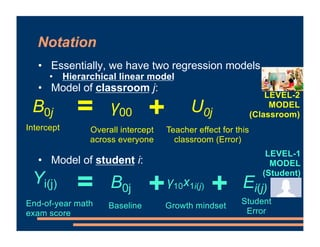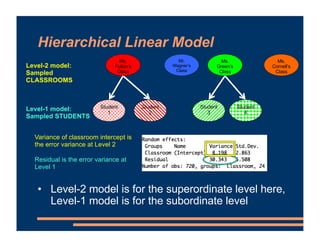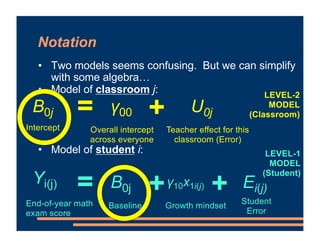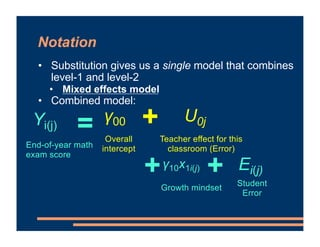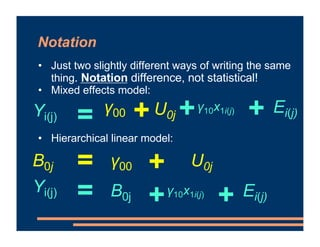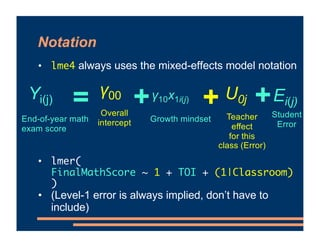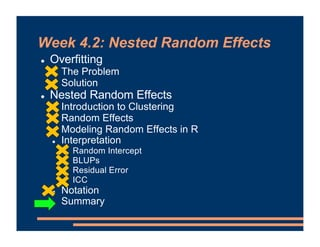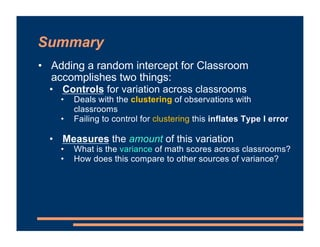1 of 79

### Mixed Effects Models - Random Intercepts

• 1. Course Business ! Next three weeks: Random effects for different types of designs ! This week and next: “Nested” random effects ! After that: “Crossed” random effects ! Informal Early Feedback survey will be available on Canvas after class ! Look under “Quizzes”
• 2. Course Business ! Package sjPlot provides a convenient way to plot lmer results ! library(sjPlot) ! model2 %>% plot_model() ! A ggplot, so all ggplot settings can be used Each row is one independent variable (or interaction) Confidence interval x-axis: Estimate of the effect (compare to 0)
• 3. Week 4.2: Nested Random Effects ! Overfitting ! The Problem ! Solution ! Nested Random Effects ! Introduction to Clustering ! Random Effects ! Modeling Random Effects in R ! Interpretation ! Random Intercept ! BLUPs ! Residual Error ! ICC ! Notation ! Summary
• 4. Overfitting • The “Madden curse”… • Each year, a top NFL football player is picked to appear on the cover of the Madden NFL video game • That player often doesn’t play as well in the following season • Is the cover “cursed”?
• 5. Overfitting • The “Madden curse”… • Each year, a top NFL football player is picked to appear on the cover of the Madden NFL video game • That player often doesn’t play as well in the following season • Is the cover “cursed”?
• 6. Overfitting • What’s needed to be one of the top NFL players in a season? • You have to be a good player • Genuine predictor (signal) • And, luck on your side • Random chance or error • Top-performing player probably very good and very lucky • The next season… • Your skill may persist • Random chance probably won’t • Regression to the mean • Madden video game cover imperfect predicts next season’s performance because it was partly based on random error
• 7. Overfitting • Our estimates (& any choice of variables based on them) always partially reflect random chance in the dataset we used to obtain them • Won’t fit any later data set quite as well … shrinkage • Problem when we’re using the data to decide the model
• 8. Overfitting • Our estimates (& any choice of variables based on them) always partially reflect random chance in the dataset we used to obtain them • Won’t fit any later data set quite as well … shrinkage • “If you use a sample to construct a model, or to choose a hypothesis to test, you cannot make a rigorous scientific test of the model or the hypothesis using that same sample data.” (Babyak, 2004, p. 414)
• 9. Overfitting—Examples • Relations that we observe between a predictor variable and a dependent variable might simply be capitalizing on random chance • U.S. government puts out 45,000 economic statistics each year (Silver, 2012) • Can we use these to predict whether US economy will go into recession? • With 45,000 predictors, we are very likely to find a spurious relation by chance • Especially w/ only 15 recessions since the end of WW II
• 10. Overfitting—Examples • Relations that we observe between a predictor variable and a dependent variable might simply be capitalizing on random chance • U.S. government puts out 45,000 economic statistics each year (Silver, 2012) • Can we use these to predict whether US economy will go into recession? • With 45,000 predictors, we are very likely to find a spurious relation by chance • Significance tests try to address this … but with 45,000 predictors, we are likely to find significant effects by chance (5% Type I error rate at ɑ=.05)
• 11. Overfitting—Examples • Adak Island, Alaska • Daily temperature here predicts stock market activity! • r = -.87 correlation with the price of a specific group of stocks! • Completely true—I’m not making this up! • Problem with this: • With thousands of weather stations & stocks, easy to find a strong correlation somewhere, even if it’s just sampling error • Problem is that this factoid doesn’t reveal all of the other (non- significant) weather stations & stocks we searched through • Would only be impressive if this hypothesis continued to be true on a new set of weather data & stock prices Vul et al., 2009
• 12. Overfitting—Examples • “Puzzlingly high correlations” in some fMRI work • Correlate each voxel in a brain scan with a behavioral measure (e.g., personality survey) • Restrict the analysis to voxels where the correlation is above some threshold • Compute final correlation in this region with behavioral measure—very high! • Problem: Voxels were already chosen based on those high correlations • Includes sampling error favoring the correlation but excludes error that doesn’t Vul et al., 2009
• 13. Week 4.2: Nested Random Effects ! Overfitting ! The Problem ! Solution ! Nested Random Effects ! Introduction to Clustering ! Random Effects ! Modeling Random Effects in R ! Interpretation ! Random Intercept ! BLUPs ! Residual Error ! ICC ! Notation ! Summary
• 14. Overfitting—Solutions • One solution: Select model(s) in advance (perhaps even pre-registered) • A theory is valuable for this • Adak Island example is implausible in part because there’s no causal reason why an island in Alaska would relate to stock prices “Just as you do not need to know exactly how a car engine works in order to drive safely, you do not need to understand all the intricacies of the economy to accurately read those gauges.” – Economic forecasting firm ECRI (quoted in Silver, 2012)
• 15. Overfitting—Solutions • One solution: Select model(s) in advance (perhaps even pre-registered) • A theory is valuable for this • Not driven purely by the data or by chance if we have an a priori reason to favor this variable “There is really nothing so practical as a good theory.” -- Social psychologist Kurt Lewin (Lewin’s Maxim)
• 16. Overfitting—Solutions • One solution: Select model(s) in advance (perhaps even pre-registered) • A theory is valuable for this • Not driven purely by the data or by chance if we have an a priori reason to favor this variable • Based on some other measure (e.g., another brain scan)
• 17. Overfitting—Solutions • One solution: Select model(s) in advance (perhaps even pre-registered) • A theory is valuable for this • Not driven purely by the data or by chance if we have an a priori reason to favor this variable • Based on some other measure (e.g., another brain scan) • Based on research design • For factorial experiments, typical to include all experimental variables and interactions • Research design implies you were interested in all of these • Variables viewed in advance as necessary controls
• 18. Overfitting—Solutions • For more exploratory analyses: Show that the finding replicates • On a second dataset • Test a model obtained from one subset of the data applies to another subset (cross-validation) • e.g., training and test sets • A better version: Do this with many randomly chosen subsets • Bootstrapping methods • Reading on Canvas for some general ways to do this in R
• 19. Overfitting—Solutions • Also: Can limit the number of variables • The more variables relative to our sample size, the more likely we are to be overfitting • Common rule of thumb (Babyak, 2004): • 10-15 observations per predictor • e.g., 4 predictor variables of interest " N=40 to 60 needed
• 20. Week 4.2: Nested Random Effects ! Overfitting ! The Problem ! Solution ! Nested Random Effects ! Introduction to Clustering ! Random Effects ! Modeling Random Effects in R ! Interpretation ! Random Intercept ! BLUPs ! Residual Error ! ICC ! Notation ! Summary
• 21. Theories of Intelligence ! For each item, rate your agreement on a scale of 0 to 7 DEFINITELY AGREE DEFINITELY DISAGREE 7 0
• 22. Theories of Intelligence 1. “You have a certain amount of intelligence, and you can’t really do much to change it.” DEFINITELY AGREE DEFINITELY DISAGREE 7 0
• 23. Theories of Intelligence 2. “Your intelligence is something about you that you can’t change very much.” DEFINITELY AGREE DEFINITELY DISAGREE 7 0
• 24. Theories of Intelligence 3. “You can learn new things, but you can’t really change your basic intelligence.” DEFINITELY AGREE DEFINITELY DISAGREE 7 0
• 25. Theories of Intelligence ! Find your total, then divide by 3 ! Learners hold different views of intelligence (Dweck, 2008): FIXED MINDSET: Intelligence is fixed. Performance = ability GROWTH MINDSET: Intelligence is malleable Performance = effort 7 0
• 26. Theories of Intelligence • Fixed mindset has been linked to less persistence & success in academic (& other work) (Dweck, 2008) • Let’s see if this is true for middle-schoolers’ math achievement • math.csv on Canvas • 30 students in each of 24 classrooms (N = 720) • Measure fixed mindset … 0 to 7 questionnaire • Dependent measure: Score on an end-of-year standardized math exam (0 to 100)
• 27. Theories of Intelligence • We can start writing a regression line to relate fixed mindset to end-of-year score = End-of-year math exam score Yi(j) Fixed mindset γ10x1i(j)
• 28. Theories of Intelligence • What about kids whose Fixed Mindset score is 0? • Completely Growth mindset • These kids probably will score decently well on the math exam • Include an intercept term • Math score when Fixed Mindset score = 0 = End-of-year math exam score + Baseline Yi(j) γ00 Fixed mindset γ10x1i(j)
• 29. Theories of Intelligence • We probably can’t predict each student’s math score exactly • Kids differ in ways other than their fixed mindset • Include an error term • Residual difference between predicted & observed score for observation i in classroom j • Captures what’s unique about child i • Assume these are independently, identically normally distributed (mean 0) Error Ei(j) = End-of-year math exam score + + Baseline Yi(j) γ00 Fixed mindset γ10x1i(j)
• 30. Theories of Intelligence Data Student 1 Student 2 Student 3 Student 4 Sampled STUDENTS Mr. Wagner’s Class Ms. Fulton’s Class Ms. Green’s Class Ms. Cornell’s Class Sampled CLASSROOMS Math achievement score y11 Theory of intelligence score x111 Independent error term e11 Math achievement score y21 Theory of intelligence score x121 Independent error term e21 Math achievement score y42 Theory of intelligence score x142 Independent error term e42 • Where is the problem here?
• 31. Theories of Intelligence Data Student 1 Student 2 Student 3 Student 4 Sampled STUDENTS Mr. Wagner’s Class Ms. Fulton’s Class Ms. Green’s Class Ms. Cornell’s Class Sampled CLASSROOMS Math achievement score y11 Theory of intelligence score x111 Independent error term e11 Math achievement score y21 Theory of intelligence score x121 Independent error term e21 Math achievement score y42 Theory of intelligence score x142 Independent error term e42 • Error terms not fully independent • Students in the same classroom probably have more similar scores. Clustering. • Differences in classroom size, teaching style, teacher’s experience…
• 32. Clustering • Why does clustering matter? • Remember that we test effects by comparing the estimates to their standard error: • Failing to account for clustering can lead us to detect spurious results (sometimes quite badly!) t = Estimate Std. error But if we have a lot of kids from the same classroom, they share more similarities than all kids in population Understating the standard error across subjects… …thus overstating the significance test
• 33. Week 4.2: Nested Random Effects ! Overfitting ! The Problem ! Solution ! Nested Random Effects ! Introduction to Clustering ! Random Effects ! Modeling Random Effects in R ! Interpretation ! Random Intercept ! BLUPs ! Residual Error ! ICC ! Notation ! Summary
• 34. Fixed Effects vs. Random Effects • 1 + TOI + Classroom What we want to know about the Classroom variable, and how we are using it, is different from the effect of Theory Of Intelligence. Can’t we just add Classroom as another fixed effect variable?
• 35. Fixed Effects vs. Random Effects • What makes the Classroom variable different from the TOI variable? # If we included Classroom as a fixed effect, we’d get many, many comparisons between individual classrooms
• 36. Fixed Effects vs. Random Effects • What makes the Classroom variable different from the TOI variable? # If we included Classroom as a fixed effect, we’d get many, many comparisons between individual classrooms # But, our theoretical interest is in effects of theories of intelligence, not in effects of being Ms. Fulton # If another researcher wanted to replicate this experiment, they could include the Theories of Intelligence scale, but they probably couldn’t get the same teachers # We do expect our results to generalize to other teachers/classrooms, but this experiment doesn’t tell us anything about how the relation would generalize to other questionnaires
• 37. Fixed Effects vs. Random Effects • What makes the Classroom variable different from the TOI variable? • These classrooms are just some classrooms we sampled out of the population of interest # Fixed effects: • We’re interested in the specific categories/levels • The categories are a complete set • At least within the context of the research design # Random effects: • Not interested in the specific categories • Observed categories are simply a sample out of a larger population
• 38. Fixed Effect or Random Effect? • Scott interested in the effects of distributing practice over time on statistics learning. For his experimental items, he picks 10 statistics formulae randomly out of a textbook. Then, he samples 20 Pittsburgh-area grad students as participants. Half study the items using distributed practice and half study using massed practice (a single day) before they are all tested. 1. Participant is a… 2. Item is a… 3. Practice type (distributed vs. massed) is a …
• 39. Fixed Effect or Random Effect? • Scott interested in the effects of distributing practice over time on statistics learning. For his experimental items, he picks 10 statistics formulae randomly out of a textbook. Then, he samples 20 Pittsburgh-area grad students as participants. Half study the items using distributed practice and half study using massed practice (a single day) before they are all tested. 1. Participant is a… • Random effect. Scott sampled them out of a much larger population of interest (grad students). 2. Item is a… • Random effect. Scott’s not interested in these specific formulae; he picked them out randomly. 3. Practice type (distributed vs. massed) is a … • Fixed effect. We’re comparing these 2 specific conditions
• 40. Fixed Effect or Random Effect? 4. A researcher in education is interested in the relation between class size and student evaluations at the university level. The research team collects data at 10 different universities across the US. University is a… 5. A planner for the city of Pittsburgh compares the availability of parking at Pitt vs CMU. University is a…
• 41. Fixed Effect or Random Effect? 4. A researcher in education is interested in the relation between class size and student evaluations at the university level. The research team collects data at 10 different universities across the US. University is a… • Random effect. Goal is to generalize to universities as a whole, and we just sampled these 10. 5. A planner for the city of Pittsburgh compares the availability of parking at Pitt vs CMU. University is a… • Fixed effect. Now, we DO care about these two particular universities.
• 42. Fixed Effect or Random Effect? 6. We’re testing the effectiveness of a new SSRI on depressive systems. In our clinical trial, we manipulate the dosage of the SSRI that participants receive to be either 0 mg (placebo), 10 mg, or 20 mg per day based on common prescriptions. Dosage is a…
• 43. Fixed Effect or Random Effect? 6. We’re testing the effectiveness of a new SSRI on depressive systems. In our clinical trial, we manipulate the dosage of the SSRI that participants receive to be either 0 mg (placebo), 10 mg, or 20 mg per day based on common prescriptions. Dosage is a… • Fixed effect. This is the variable that we’re theoretically interested in and want to model. Also, 0, 10, and 20 mg exhaustively characterize dosage within this experimental design.
• 44. Week 4.2: Nested Random Effects ! Overfitting ! The Problem ! Solution ! Nested Random Effects ! Introduction to Clustering ! Random Effects ! Modeling Random Effects in R ! Interpretation ! Random Intercept ! BLUPs ! Residual Error ! ICC ! Notation ! Summary
• 45. Modeling Random Effects • Let’s add Classroom as a random effect to the model • model1 <- lmer(FinalMathScore ~ 1 + TOI + (1|Classroom), data=math) • We are now controlling for some classrooms having higher scores than others • Still a significant TOI effect!
• 46. Modeling Random Effects • What is (1|Classroom) doing? • model1 <- lmer(FinalMathScore ~ 1 + TOI + (1|Classroom), data=math) • We’re allowing each classroom to have a different intercept • Some classrooms have higher math scores on average • Some have lower math scores on average • A random intercept
• 47. Modeling Random Effects • What is (1|Classroom) doing? • model1 <- lmer(FinalMathScore ~ 1 + TOI + (1|Classroom), data=math) • We are not interested in comparing the specific classrooms we sampled • Instead, we are model the variance of this population • How much do classrooms typically vary in math achievement?
• 48. Modeling Random Effects • Model results: • We are not interested in comparing the specific classrooms we sampled • Instead, we are model the variance of this population • How much do classrooms typically vary in math achievement? • Standard deviation across classrooms is 2.86 points Additional, unexplained subject variance (even after accounting for classroom differences) Variance of classroom intercepts
• 49. Week 4.2: Nested Random Effects ! Overfitting ! The Problem ! Solution ! Nested Random Effects ! Introduction to Clustering ! Random Effects ! Modeling Random Effects in R ! Interpretation ! Random Intercept ! BLUPs ! Residual Error ! ICC ! Notation ! Summary
• 50. Understanding the Random Intercept ! Think back to a normal distribution… ! The standard normal has mean 0 and standard deviation 1 1
• 51. Understanding the Random Intercept ! We can also have normal distributions with other means and standard deviations ! This one has mean ~66 and standard deviation ~3 3
• 52. Understanding the Random Intercept ! Fixed intercept tells us that the mean intercept, across all classes, is 66
• 53. Understanding the Random Intercept ! But, there is a distribution of class averages ! Some classrooms average high or lower than that ! This distribution has a standard deviation of ~2.9 (std. deviation of random intercept) 3 64% of classrooms have an intercept between 63 and 69 96% of classrooms have an intercept between 60 and 72
• 54. Understanding the Random Intercept ! So: ! Fixed intercept tell us the mean of the distribution: 66 ! Standard deviation of the random intercept tells us the standard deviation of that distribution: 2.9 ! Assumed in lmer() to be a normal distribution 3 64% of classrooms have an intercept between 63 and 69 96% of classrooms have an intercept between 60 and 72
• 55. Understanding the Random Intercept ! Our classroom are a random sample from this population of classrooms with different class averages 3 64% of classrooms have an intercept between 63 and 69 96% of classrooms have an intercept between 60 and 72
• 56. Understanding the Random Intercept ! How much variance tells us how much variability there is across classrooms ! i.e., how wide a spread of classrooms ! e.g., if the SD had only been 1 " less variable 64% of classrooms have an intercept between 65 and 67 96% of classrooms have an intercept between 64 and 68
• 57. Understanding the Random Intercept ! How much variance tells us how much variability there is across classrooms ! i.e., how wide a spread of classrooms ! Or if the SD had been 10 " much more variable 64% of classrooms have an intercept between 56 and 76 96% of classrooms have an intercept between 46 and 86
• 58. Caveats • For a fair estimate of the population variance: • At least 5-6 clustering units, 10+ preferred (e.g., 5+ classrooms) (Bolker, 2018) • Population size is at least 100x the number of groups you have (e.g., at least 2400 classrooms in the world) (Smith, 2013) • If not, should still include the random effect to account for clustering. Just wouldn’t a good estimate of the population variance • For a true “random effect”, the observed set of categories samples from a larger population • If we’re not trying to generalize to a population, might instead call this a variable intercept model (Smith, 2013)
• 59. Week 4.2: Nested Random Effects ! Overfitting ! The Problem ! Solution ! Nested Random Effects ! Introduction to Clustering ! Random Effects ! Modeling Random Effects in R ! Interpretation ! Random Intercept ! BLUPs ! Residual Error ! ICC ! Notation ! Summary
• 60. BLUPs ! Where do individual classrooms fall in this distribution? • ranef(model1) • Shows you the intercepts for individual classrooms • These are adjustments relative to the fixed effect • Best Linear Unbiased Predictors (BLUPs) Ms. Baker’s classroom has a class average that is +4.5 relative to the overall intercept Mean intercept is 66 ms, so intercept for Ms. Baker’s class: 66 + 4.5 = 70.5
• 61. BLUPs • Why aren’t these BLUPs displayed in our initial results from summary()? • For random effects, we’re mainly interested in modeling variability • BLUPs aren’t considered parameters of the model • Not what this is a model “of” • We ran this analysis to model the effects of TOI on kids’ math performance, not the effect of being Ms. Baker from Allentown • If we ran the same design with a different sample, BLUPs probably wouldn’t be the same • No reason to expect that Classroom #12 in the new sample will again be one of the better classrooms • By contrast, we do intend for our fixed effects to replicate
• 62. Week 4.2: Nested Random Effects ! Overfitting ! The Problem ! Solution ! Nested Random Effects ! Introduction to Clustering ! Random Effects ! Modeling Random Effects in R ! Interpretation ! Random Intercept ! BLUPs ! Residual Error ! ICC ! Notation ! Summary
• 63. Residual Variance • We now know how to understand the Classroom variance • What about the Residual variance? • This is the variance of the residuals • Variance in individual math scores not explained by any of our other variables: • Overall intercept • Theory of intelligence • Classroom differences • True error variance • In this case, what’s unique about child i
• 64. Residual Variance ! There is a distribution of child-level residuals ! This distribution has a standard deviation of 5.5 ! Mean of the distribution of residuals is 0 by definition 5.5 64% of children have a residual between -5.5 and 5.5 96% of children have a residual between -11 and 11
• 65. Week 4.2: Nested Random Effects ! Overfitting ! The Problem ! Solution ! Nested Random Effects ! Introduction to Clustering ! Random Effects ! Modeling Random Effects in R ! Interpretation ! Random Intercept ! BLUPs ! Residual Error ! ICC ! Notation ! Summary
• 66. Intraclass Correlation Coefficient • Model results: • The intraclass correlation coefficient measures how much variance is attributed to a particular random effect ICC = Variance of Random Effects of Interest Sum of All Random Effect Variances = Classroom Variance Classroom Variance + Residual Variance ≈ .21
• 67. Intraclass Correlation Coefficient • The intraclass correlation coefficient measures how much variance is attributed to a random effect • Proportion of all random variation that has to do with classrooms • 21% of random student variation due to which classroom they are in • Also the correlation among observations from the same classroom • High correlation among observations from the same classroom = Classroom matters a lot = high ICC • Low correlation among observations from the same classroom = Classroom not that important = low ICC
• 68. Week 4.2: Nested Random Effects ! Overfitting ! The Problem ! Solution ! Nested Random Effects ! Introduction to Clustering ! Random Effects ! Modeling Random Effects in R ! Interpretation ! Random Intercept ! BLUPs ! Residual Error ! ICC ! Notation ! Summary
• 69. Notation • What exactly is this model doing? • Let’s go back to our model of individual students (now slightly different): Student Error Ei(j) = End-of-year math exam score + + Baseline Yi(j) B0j Fixed mindset γ10x1i(j)
• 70. Notation • What exactly is this model doing? • Let’s go back to our model of individual students (now slightly different): Student Error Ei(j) = End-of-year math exam score + + Baseline Yi(j) B0j Fixed mindset γ10x1i(j) What now determines the baseline that we should expect for students with fixed mindset=0?
• 71. Notation • What exactly is this model doing? • Baseline (intercept) for a student in classroom j now depends on two things: • Let’s go back to our model of individual students (now slightly different): Student Error Ei(j) = End-of-year math exam score + + Baseline Yi(j) B0j Fixed mindset γ10x1i(j) U0j = Intercept + Overall intercept across everyone B0j γ00 Teacher effect for this classroom (Error)
• 72. Notation • Essentially, we have two regression models • Hierarchical linear model • Model of classroom j: • Model of student i: Student Error Ei(j) = End-of-year math exam score + + Baseline Yi(j) B0j Growth mindset γ10x1i(j) U0j = Intercept + B0j γ00 Teacher effect for this classroom (Error) LEVEL-1 MODEL (Student) LEVEL-2 MODEL (Classroom) Overall intercept across everyone
• 73. Hierarchical Linear Model Student 1 Student 2 Student 3 Student 4 Level-1 model: Sampled STUDENTS Mr. Wagner’s Class Ms. Fulton’s Class Ms. Green’s Class Ms. Cornell’s Class Level-2 model: Sampled CLASSROOMS • Level-2 model is for the superordinate level here, Level-1 model is for the subordinate level Variance of classroom intercept is the error variance at Level 2 Residual is the error variance at Level 1
• 74. Notation • Two models seems confusing. But we can simplify with some algebra… • Model of classroom j: • Model of student i: Student Error Ei(j) = End-of-year math exam score + + Baseline Yi(j) B0j Growth mindset γ10x1i(j) U0j = Intercept + B0j γ00 Teacher effect for this classroom (Error) LEVEL-1 MODEL (Student) LEVEL-2 MODEL (Classroom) Overall intercept across everyone
• 75. Notation • Substitution gives us a single model that combines level-1 and level-2 • Mixed effects model • Combined model: Student Error Ei(j) = End-of-year math exam score + + Yi(j) Growth mindset γ10x1i(j) U0j + Overall intercept γ00 Teacher effect for this classroom (Error)
• 76. Notation • Just two slightly different ways of writing the same thing. Notation difference, not statistical! • Mixed effects model: • Hierarchical linear model: Ei(j) = + + Yi(j) γ10x1i(j) U0j + γ00 Ei(j) = Yi(j) B0j γ10x1i(j) U0j = + B0j γ00 + +
• 77. Notation • lme4 always uses the mixed-effects model notation • lmer( FinalMathScore ~ 1 + TOI + (1|Classroom) ) • (Level-1 error is always implied, don’t have to include) Student Error Ei(j) = End-of-year math exam score + + Yi(j) Growth mindset γ10x1i(j) U0j + Overall intercept γ00 Teacher effect for this class (Error)
• 78. Week 4.2: Nested Random Effects ! Overfitting ! The Problem ! Solution ! Nested Random Effects ! Introduction to Clustering ! Random Effects ! Modeling Random Effects in R ! Interpretation ! Random Intercept ! BLUPs ! Residual Error ! ICC ! Notation ! Summary
• 79. Summary • Adding a random intercept for Classroom accomplishes two things: • Controls for variation across classrooms • Deals with the clustering of observations with classrooms • Failing to control for clustering this inflates Type I error • Measures the amount of this variation • What is the variance of math scores across classrooms? • How does this compare to other sources of variance?